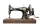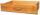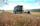Observatory

Observatory dome has the shape of a hemisphere with a diameter d = 10 m. Calculate the surface.

Result

S =  157.1 m2

Solution:Leave us a comment of example and its solution (i.e. if it is still somewhat unclear...):Be the first to comment!Next similar examples:

1. Half-sphere roofThe roof above the castle tower has the shape of a 12.8 m diameter half-sphere. What is the cost of this roof, if the cost of 1 square meter is 12 euros and 40 cents?
2. GreenhouseGarden plastic greenhouse is shaped half cylinder with a diameter of 6 m and base length 20 m. At least how many m2 of plastic is need to its cover?
3. PipeHow long is the pipe with an outside diameter of 1.44 m if his coloring consumed 44 kg of color. 1 kg of color coverage is 9 m2.
4. Fit ballWhat is the size of the surface of Gymball (FIT - ball) with a diameter of 65 cm?
5. AverageThe arithmetic mean of the two numbers is 71.7. One number is 5. Calculate the second number.
6. RapeThe agricultural cooperative harvested 525 ares of rape, of which received 5.6 tons of rape seeds. Calculate the yield per hectare of rape.
7. Sphere VSFind the surface and volume of a sphere that has a radius of 2 dm.
8. BasenHow many square meters of tiles we need to tile the walls and floor of the pool 15 meters long, six meters wide and two meters?deep
9. GardenThe garden around the new majer is divided as follows: 35% vegetable, 30% fruit orchards, 10% flowers and the remaining 120 m2 are lawns. What is the total area of the garden?Combine like terms 4c+c-7c
11. SewingThe lady cut off one half of cloth. She needed three-quarters of this piece to sew a skirt. What part of the original piece of cloth still remained?
12. Seeds 2How many seeds germinated from 1000 pcs, when 23% no emergence?
13. DrawerThe rectangular face of a drawer has a perimeter of 108 cm and a width of 18cm. Find the length and the area of the rectangular face of the drawer.
14. IronIron ore contains 57% iron. How much ore is needed to produce 20 tons of iron?
15. FarmlandFarmers seeded maize to 45 ha, which is 15% of their total farmland. How many hectares of fields they have?
16. Obtuse angleWhich obtuse angle is creating clocks at 17:00?
17. ClassIn 7.C clss are 10 girls and 20 boys. Yesterday was missing 20% of girls and 50% boys. What percentage of students missing?